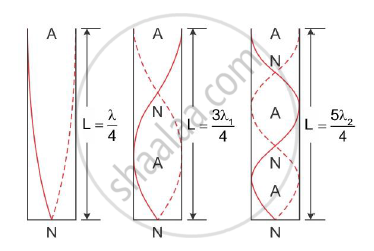HSC Science (Computer Science) 12th Board ExamMaharashtra State Board
Share

Show that Only Odd Harmonics Are Present in an Air Column Vibrating in a Pipe Closed at One End. - HSC Science (Computer Science) 12th Board Exam - Physics

Question

Show that only odd harmonics are present in an air column vibrating in a pipe closed at one end.

Solution

Consider the different modes of vibration of an air column within a pipe closed at one end. Let L be the length of the pipe.

Stationary waves are formed within the air column when the time taken by the sound waves to produce a compression and rarefaction becomes equal to the time taken by the wave to travel twice the length of the tube. The standing waves are formed only for certain discrete frequencies.In the first mode of vibration of the air column, there is one node and one antinode as shown in the figure above.

If λ is the length of the wave in the fundamental mode of vibration,

Then,Length of the air column,L=λ/4

⇒ λ = 4L .................... (1)

As velocity of the wave, v = nλ

n = v/λ

substituting (1) we get:

n = v/4L; Frequency of the fundamental mode

In the second mode of vibration of the air column, two nodes and two antinodes are formed.

In this case:

The length of the air column, L=3λ1/4

where λ1 is the wavelength of the wave in the second mode of vibration,

⇒λ = 4L/3 .................(1)

v = n1λ1

n1 = v/λ1

substituting (1), we get

n_1="v"/((4L)/3)=(3"v")/(4L)=3"n"

This frequency is called the third harmonics or first overtone

Similarly, during the third mode of vibration of the air column, three nodes and three antinodes are formed.

Here,

The length of the air column, L = 5λ2/4

where λ2 is the wavelength of the wave in third mode of vibration,

⇒ λ2 = 4L/5 ...........................(1)

v = n2λ2

n2 = v/λ2

substituting (1), we get

n_2 ="v"/((4L)/5)=(5"v")/(4L)=5"n"

This frequency is called the fifth harmonic or second overtone.

Thus, we see that the frequencies of the modes of vibrations are in the ratio n:n1:n2 = 1:3:5. This shows that only odd harmonics are present in the modes of vibrations of the air column closed at one end.

Is there an error in this question or solution?

APPEARS IN

2014-2015 (March) (with solutions)
Question 4.1 | 7.00 marks

Video TutorialsVIEW ALL 

Solution Show that Only Odd Harmonics Are Present in an Air Column Vibrating in a Pipe Closed at One End. Concept: Free and Forced Vibrations.
S